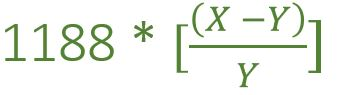Related Articles

# Program to find the Speed of train as per speed of sound

• Last Updated : 08 Apr, 2021

Two guns were fired from same place at an interval of ‘X’ minutes. A person who is approaching the shooting place by train hears the shooting sound at an interval of ‘Y’ minutes, The task is to find the ‘S’ i.e speed of the train.
Note: Speed of the sound in air is 330m/s in a fixed value.

Examples:

Input: X = 14 min, Y = 13.5 min
Output: S = 44 km/hr

Input: X = 8 min, Y = 7.2 min
Output: S = 132 km/hr

Approach: Take the first example,

• Distance covered by the train in Y minutes
= Distance covered by the sound in (X – Y) minutes
= 330 * (X – Y) * 60 minutes (Distance = speed of sound * time)
• Speed of the train
= (330 * (X – Y) * 60 ) / Y (Speed = distance / time)
= (330 * 60 * (X – Y)) / 1000 * (60 / y)
= 1188 [ (X – Y) / Y] km/hr
• Now, According to 1st example
Time difference i.e (X – Y) = 14.00 – 13.30 = 30sec
Speed of train = 1188 * [ (14 – 13.5) / 13.5 ] = 44km/hr
• This can be calculated by using the below formula:Below is the implementation of the above approach.

## C++

 `// C++ implementation of the approach` `#include ``using` `namespace` `std;` `// Function to find the``// Speed of train``float` `speedOfTrain(``float` `X, ``float` `Y)``{` `    ``float` `Speed = 0;` `    ``Speed = 1188 * ((X - Y) / Y);` `    ``return` `Speed;``}` `// Driver code``int` `main()``{``    ``float` `X = 8, Y = 7.2;` `    ``// calling Function``    ``cout << speedOfTrain(X, Y)``         ``<< ``" km/hr"``;` `    ``return` `0;``}`

## Java

 `// Java implementation of the approach``class` `GFG``{` `    ``// Function to find the``    ``// Speed of train``    ``static` `int` `speedOfTrain(``float` `X, ``float` `Y)``    ``{``        ``float` `Speed;` `        ``Speed = ``1188` `* ((X - Y) / Y);` `        ``return` `(``int``)Speed;``    ``}` `    ``// Driver code``    ``public` `static` `void` `main(String[] args)``    ``{``        ``float` `X = 8f, Y = ``7``.2f;` `        ``// calling Function``        ``int` `result = (speedOfTrain(X, Y));``        ``System.out.println(result + ``" km/hr"``);``    ``}``}` `// This code is contributed by PrinciRaj1992`

## Python3

 `# Python3 implementation of the approach``from` `math ``import` `ceil` `# Function to find the``# Speed of train``def` `speedOfTrain(X, Y):``    ``Speed ``=` `0` `    ``Speed ``=` `1188` `*` `((X ``-` `Y) ``/` `Y)` `    ``return` `Speed` `# Driver code``if` `__name__ ``=``=` `'__main__'``:``    ``X ``=` `8``    ``Y ``=` `7.2` `    ``# calling Function``    ``print``(ceil(speedOfTrain(X, Y)),``                   ``end ``=` `" km/hr"``)` `# This code is contributed by``# Surendra_Gangwar`

## C#

 `// C# implementation of the approach``using` `System;` `class` `GFG``{` `    ``// Function to find the``    ``// Speed of train``    ``static` `int` `speedOfTrain(``float` `X, ``float` `Y)``    ``{``        ``float` `Speed;` `        ``Speed = 1188 * ((X - Y) / Y);` `        ``return` `(``int``)Speed;``    ``}` `    ``// Driver code``    ``public` `static` `void` `Main()``    ``{``        ``float` `X = 8f, Y = 7.2f;` `        ``// calling Function``        ``int` `result = (speedOfTrain(X, Y));``        ``Console.Write(result + ``" km/hr"``);``    ``}``}` `// This code is contributed by anuj_67..`

## Javascript

 ``
Output:
`132 km/hr`

Attention reader! Don’t stop learning now. Get hold of all the important DSA concepts with the DSA Self Paced Course at a student-friendly price and become industry ready.  To complete your preparation from learning a language to DS Algo and many more,  please refer Complete Interview Preparation Course.

In case you wish to attend live classes with experts, please refer DSA Live Classes for Working Professionals and Competitive Programming Live for Students.

My Personal Notes arrow_drop_up